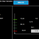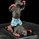FX:XAUUSD   Золото / Доллар США
1858 просмотров
1858
Hello.
By requests I saved myself some free time and can publish this small insight of how I analyse market by new dynamic level theory, what means you put SL on the trade you take right where trend is to change.
This looks like matrix and I cannot tell all 5 year work in just one Idea as it takes big time to understand.
But I will try to show you the vision of how I analyse market and why I get target 1193 - 1180

Don't pay attention to level names MDCIP etc etc.. that's for me.
Of course this process for me is automated as machine / software calculates this all levels for me, but this is how it looks if you do it manually.

So we start from Yearly Dynamic level MDCIP. And once it is broken you take trend angle tool and draw it from broken place up to last high. Then from last High you draw the same back to last low.
MDCIP Constant degree number is 118.28 ( 28 is always added because 28 is constant gold number and represent the source of formula )
So H degree and L degree cannot be greater than this number, if this is greater then behaviour in market will change and new MDCIP level is created, if we count -111 + 88 you see that the number is not greater than 118.28 so therefore we know that MDCIP is still a constant level.

Once we done that we take closest Alteration level which by formulas and calculated leves is 2017 / 02 / 02. MMPD that represent two numbers 66 / 57. We know that MMPD is 1239.97.
So this is a new dynamic source level and we take the same tool from that date and price and draw to last High affiliated with MDCIP.
The number we get is 136. So we need to take 136 divide it by 66 which is 2.06 then we add 28 to it which is 30.06
Now we take 30.06 and divide it by 118.28 which is 2.29 and we add 0.57 to it which is 2.86.
Now we know that related PV level ( Constant Permanent Volume level ) is 1180 so we + that to 2.86 which is .
1182.26 and this time add 0.28 to it not 28 which is 1182.54.
PV level 1180 represents MPI ( Monthly Permanent inclination ) and two related pivots to it is 0.66 and 0.78.
So we take degree tool from 1180 but draw it to the last low after close and we get number 162.
( we use the same date 2017 / 02 / 02.
So we take 162 divide it by 28 which is 5.78. we count off from this number MPI 0.66 which is 5.12 and then divide this by MPI 0.78 which is 4.93
Add 4.93 to 1182.54 is 1187.47 add 0.28 to it which is 1187.75.
Then last operation to take is Count MMPD pivots and MPI pivots together so 0.66 + 0.57 + 0.66 + 0.78 is 2.67
So 1187.75 + 2.67 is 1190.42 + 0.28 = 1190.70.
By unwriten rule you always + / - 3\$ on gold so this is 1193

This is enough as anyway it is a matrix for you.. but this is what my analysis are going thru!
It involves a lot more calculation to get all these pivots and numbers and levels, but I showd you just 0.00000001% of what is going on in this calculation world.
Like I said all levels are calculated by custom created machine as it would be nightmare to do it manually, but this is what machine is calculating on daily bases every day to project long term, mid term and daily targets.
Software consists of 790k lines of instruction code.
This is to answer just a little bit why 1193 is what I target.. and that could be reached, but there are a lot of rules and invalidation levels.. so we need to check when it is time to buy as we can do so without waiting 1193.
Cheers guys, appreciate your support!
TPP
Комментарий: As well to mention, I learn this all so far in 5 years non stop, and I can tell that I know maybe approx 8% at best 10% of the whole information required. So you get a hint of what is going on here.
Комментарий: Sonn will try to do some dynamic analysis again and see what comes out.Nov 29 The velocity of golds fall over the last 3 weeks puts my down side target in agreement with yours TPP at around the \$1100 level
Based on price expansion from 1377 and 1248 levels of last few months.
and if the Trump bears really push it we could see a double bottom at last December's lows of 1050 time will reveal all
ОтветитьGingermeggs
@Gingermeggs, Where did I say I have donwnside targets?
ОтветитьGingermeggs
@Gingermeggs, I am in heavy longs with Targets 1383 > 1430
Good Luck.
ОтветитьWell that is some maths tpp and from being a bobcat driver I take my hat of to you, only wish I didn't work so much do could follow trades a bit more to get profit than entrying late haha my issues not yours though keep up the amazing work
Ответить